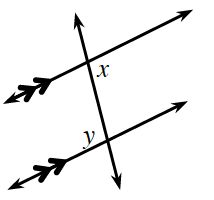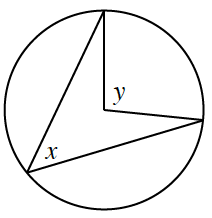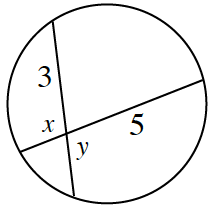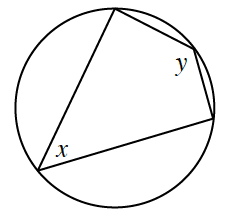### Home > CCG > Chapter Ch10 > Lesson 10.2.2 > Problem10-78

10-78.

For each diagram below, write an equation to represent the relationship between $x\text{ and }y$.

1.What is the relationship between angles created by parallel lines?

$x = y$

1.What is the relationship between central and inscribed angles?

1.Look at the triangles formed by the chords. How are they similar? How can this help you?

$3y = 5x$

1.Look at the inscribed angles. What is their relationship to the arc? How can this help you?

Recall that the measure for inscribed angles is half the arc it intercepts.

$x+y=180°$